Paul's Online Notes
Home / Calculus I / Applications of Derivatives / The Shape of a Graph, Part I
Show Mobile Notice Show All Notes Hide All Notes
Mobile Notice
You appear to be on a device with a "narrow" screen width (i.e. you are probably on a mobile phone). Due to the nature of the mathematics on this site it is best views in landscape mode. If your device is not in landscape mode many of the equations will run off the side of your device (should be able to scroll to see them) and some of the menu items will be cut off due to the narrow screen width.
Assignment Problems Notice
Please do not email me to get solutions and/or answers to these problems. I will not give them out under any circumstances nor will I respond to any requests to do so. The intent of these problems is for instructors to use them for assignments and having solutions/answers easily available defeats that purpose.

If you are looking for some problems with solutions you can find some by clicking on the "Practice Problems" link above.

### Section 4.5 : The Shape of a Graph, Part I

For problems 1 – 4 the graph of a function is given. Determine the intervals on which the function increases and decreases.

1.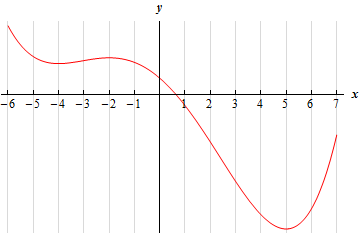2.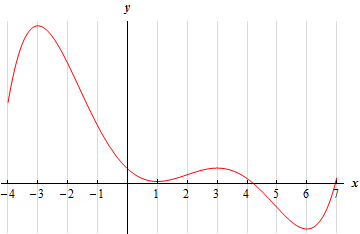3.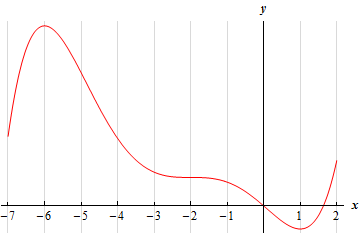4.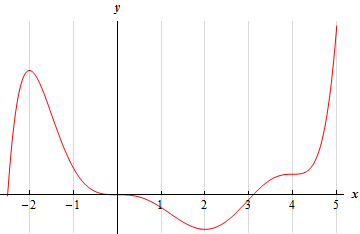For problems 5 – 7 the graph of the derivative of a function is given. From this graph determine the intervals in which the function increases and decreases.

1.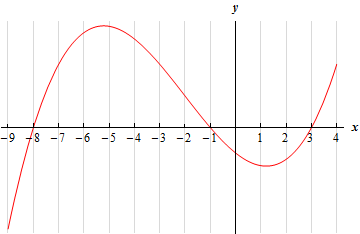2.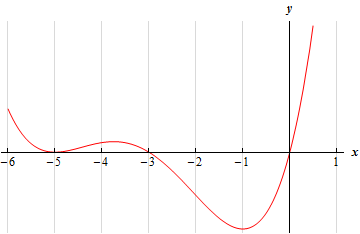3.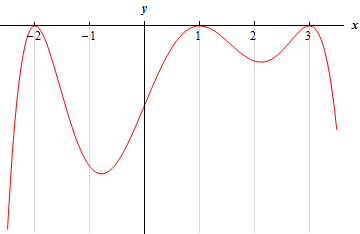For problems 8 – 10 The known information about the derivative of a function is given. From this information answer each of the following questions.

1. Identify the critical points of the function.
2. Determine the intervals on which the function increases and decreases.
3. Classify the critical points as relative maximums, relative minimums or neither.

1. $\begin{array}{c}f'\left( 1 \right) = 0\hspace{0.5in}f'\left( 3 \right) = 0\hspace{0.5in}f'\left( 8 \right) = 0\\ f'\left( x \right) < 0\,\,\,\,\,{\mbox{on}}\,\,\,\,\,\left( { - \infty ,1} \right),\,\,\,\left( {3,8} \right)\hspace{0.25in}f'\left( x \right) > 0\,\,\,\,\,{\mbox{on}}\,\,\,\,\,\left( {1,3} \right),\,\,\,\left( {8,\infty } \right)\end{array}$
2. $\begin{array}{c}g'\left( { - 2} \right) = 0\hspace{0.5in}\,g'\left( 0 \right) = 0\hspace{0.5in}g'\left( 3 \right) = 0\hspace{0.5in}\,g'\left( 6 \right) = 0\\ g'\left( x \right) < 0\,\,\,\,\,{\mbox{on}}\,\,\,\,\,\left( {0,3} \right),\,\,\,\left( {6,\infty } \right)\hspace{0.5in}g'\left( x \right) > 0\,\,\,\,\,{\mbox{on}}\,\,\,\,\,\left( { - \infty , - 2} \right),\,\,\,\left( { - 2,0} \right),\,\,\,\left( {3,6} \right)\end{array}$
3. $\begin{array}{c}h'\left( { - 1} \right) = 0\hspace{0.5in}\,h'\left( 2 \right) = 0\hspace{0.5in}h'\left( 5 \right) = 0\\ h'\left( x \right) < 0\,\,\,\,\,{\mbox{on}}\,\,\,\,\,\left( { - \infty , - 1} \right),\,\,\,\left( { - 1,2} \right)\hspace{0.5in}h'\left( x \right) > 0\,\,\,\,\,{\mbox{on}}\,\,\,\,\,\left( {2,5} \right),\,\,\,\left( {5,\infty } \right)\end{array}$

For problems 11 – 28 answer each of the following.

1. Identify the critical points of the function.
2. Determine the intervals on which the function increases and decreases.
3. Classify the critical points as relative maximums, relative minimums or neither.

1. $$f\left( t \right) = {t^3} - 15{t^2} + 63t + 3$$
2. $$g\left( x \right) = 20 + 8{x^2} + 4{x^3} - {x^4}$$
3. $$Q\left( w \right) = 8{w^3} - 18{w^2} - 24w - 10$$
4. $$\displaystyle f\left( x \right) = {x^5} + \frac{5}{4}{x^4} - 20{x^3} - 7$$
5. $$P\left( x \right) = 5 - 4x - 9{x^2} - 3{x^3}$$
6. $$R\left( z \right) = {z^5} + {z^4} - 6{z^3} + 5$$
7. $$h\left( z \right) = 1 - 12{z^2} - 9{z^3} - 2{z^4}$$
8. $$Q\left( t \right) = 7 - t + \sin \left( {4t} \right)$$on $$\displaystyle \left[ - \frac{3}{2},\frac{3}{2} \right]$$
9. $$\displaystyle f\left( z \right) = 6z - 20\cos \left( \frac{z}{2} \right)$$ on $$\left[ {0,22} \right]$$
10. $$\displaystyle g\left( x \right) = 24\cos \left( \frac{x}{3} \right) + 8x + 2$$ on $$\left[ { - 30,25} \right]$$
11. $$h\left( w \right) = 9w - 5\sin \left( {2w} \right)$$ on $$\left[ { - 5,0} \right]$$
12. $$h\left( x \right) = \sqrt{x}\,\,\left( {x + 7} \right)$$
13. $$W\left( z \right) = \left( {10 - {w^2}} \right){\left( {w + 2} \right)^{\frac{2}{3}}}$$
14. $$f\left( t \right) = \left( {{t^2} - 8} \right)\,\,\,\,\sqrt{{{t^2} - 4}}$$
15. $$f\left( x \right) = {{\bf{e}}^{\frac{1}{3}{x^{\,3}} - {x^{\,2}} - 3x}}$$
16. $$h\left( z \right) = \left( {{z^2} - 8} \right){{\bf{e}}^{3\, - z}}$$
17. $$A\left( t \right) = \ln \left( {{t^2} + 5t + 8} \right)$$
18. $$g\left( x \right) = x - 3 + \ln \left( {1 + x + {x^2}} \right)$$
19. Answer each of the following questions.
1. What is the minimum degree of a polynomial that has exactly one relative extrema?
2. What is the minimum degree of a polynomial that has exactly two relative extrema?
3. What is the minimum degree of a polynomial that has exactly three relative extrema?
4. What is the minimum degree of a polynomial that has exactly $$n$$ relative extrema?
20. For some function, $$f\left( x \right)$$, it is known that there is a relative minimum at $$x = - 4$$. Answer each of the following questions about this function.
1. What is the simplest form that the derivative of this function?
Note : There really are many possible forms of the derivative so to make the rest of this problem as simple as possible you will want to use the simplest form of the derivative.
2. Using your answer from (a) determine the most general form that the function itself can take.
3. Given that $$f\left( { - 4} \right) = 6$$ find a function that will have a relative minimum at $$x = - 4$$.
Note : There are many possible answers here so just give one of them.
21. For some function, $$f\left( x \right)$$, it is known that there is a relative maximum at $$x = - 1$$. Answer each of the following questions about this function.
1. What is the simplest form that the derivative of this function?
Note : There really are many possible forms of the derivative so to make the rest of this problem as simple as possible you will want to use the simplest form of the derivative.
2. Using your answer from (a) determine the most general form that the function itself can take.
3. Given that $$f\left( { - 1} \right) = 3$$ find a function that will have a relative maximum at $$x = - 1$$.
Note : There are many possible answers here so just give one of them.
22. For some function, $$f\left( x \right)$$, it is known that there is a critical point at $$x = 3$$ that is neither a relative minimum or a relative maximum. Answer each of the following questions about this function.
1. What is the simplest form that the derivative of this function?
Note : There really are many possible forms of the derivative so to make the rest of this problem as simple as possible you will want to use the simplest form of the derivative.
2. Using your answer from (a) determine the most general form that the function itself can take.
3. Given that $$f\left( 3 \right) = 2$$ find a function that will have a critical point at $$x = 3$$ that is neither a relative minimum or a relative maximum.
Note : There are many possible answers here so just give one of them.
23. For some function, $$f\left( x \right)$$, it is known that there is a relative maximum at $$x = 1$$ and a relative minimum at $$x = 4$$. Answer each of the following questions about this function.
1. What is the simplest form that the derivative of this function?
Note : There really are many possible forms of the derivative so to make the rest of this problem as simple as possible you will want to use the simplest form of the derivative.
2. Using your answer from (a) determine the most general form that the function itself can take.
3. Given that $$f\left( 1 \right) = 6$$ and $$f\left( 4 \right) = - 2$$ find a function that will have a relative maximum at $$x = 1$$ and a relative minimum at $$x = 4$$.
Note : There are many possible answers here so just give one of them.
24. Given that $$f\left( x \right)$$ and $$g\left( x \right)$$ are increasing functions will $$h\left( x \right) = f\left( x \right) - g\left( x \right)$$ always be an increasing function? If so, prove that $$h\left( x \right)$$ will be an increasing function. If not, find increasing functions, $$f\left( x \right)$$ and $$g\left( x \right)$$, so that $$h\left( x \right)$$ will be a decreasing function and find a different set of increasing functions so that $$h\left( x \right)$$ will be an increasing function.
25. Given that $$f\left( x \right)$$ is an increasing function. There are several possible conditions that we can impose on $$g\left( x \right)$$ so that $$h\left( x \right) = f\left( x \right) - g\left( x \right)$$ will be an increasing function. Determine as many of these possible conditions as you can.
26. For a function $$f\left( x \right)$$ determine a set of conditions on $$f\left( x \right)$$, different from those given in #15 in the practice problems, for which $$h\left( x \right) = {\left[ {f\left( x \right)} \right]^2}$$ will be an increasing function.
27. For a function $$f\left( x \right)$$ determine a single condition on $$f\left( x \right)$$ for which $$h\left( x \right) = {\left[ {f\left( x \right)} \right]^3}$$ will be an increasing function.
28. Given that $$f\left( x \right)$$ and $$g\left( x \right)$$ are positive functions. Determine a set of conditions on them for which $$h\left( x \right) = f\left( x \right)g\left( x \right)$$ will be an increasing function. Note that there are several possible sets of conditions here but try to determine the “simplest” set of conditions.
29. Repeat #38 for $$\displaystyle h\left( x \right) = \frac{{f\left( x \right)}}{{g\left( x \right)}}$$.
30. Given that $$f\left( x \right)$$ and $$g\left( x \right)$$ are increasing functions prove that $$h\left( x \right) = f\left( {g\left( x \right)} \right)$$ will also be an increasing function.